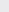Course Content

0/1

0/3

0/5

0/3

0/2

0/4

0/2

0/1

0/5

7.3 Parametric Equations and Locus 参数方程式与轨迹

(1)
Find the equation of the locus of the centers of circles passing through the points of intersection of the circle x+ y– 2x – 4y – 11 = 0 and the line y = x + 3.

x + y = 3
(2)
A variable circle touches the y-axis, and also touches a fixed circle x+ y– 8x + 2y + 13 = 0 externally. Find the equation of the locus of the centre of the circle.

y– 12x + 2y + 13 = 0
(3)
Find the equation of the locus of the centers of the circles that orthogonal with x+ y– 6x + 4y + 5 = 0 and x+ y+ 2x – 9 = 0.

4x – 2y – 7 = 0
(4)
The equation x+ y+ ax + by + 7 = 0 represents a unit circle. Find the equation of the locus of its centre as a, b vary.

x+ y= 8
A straight line through the point (0,1) meets the circle C : x+ y– 2x – 4y + 4 = 0 at points P, Psuch that the slope of PPis k. Find the equation of the locus of the mid-point of PP, as k varies.
x+ y– x – 3y + 2 = 0